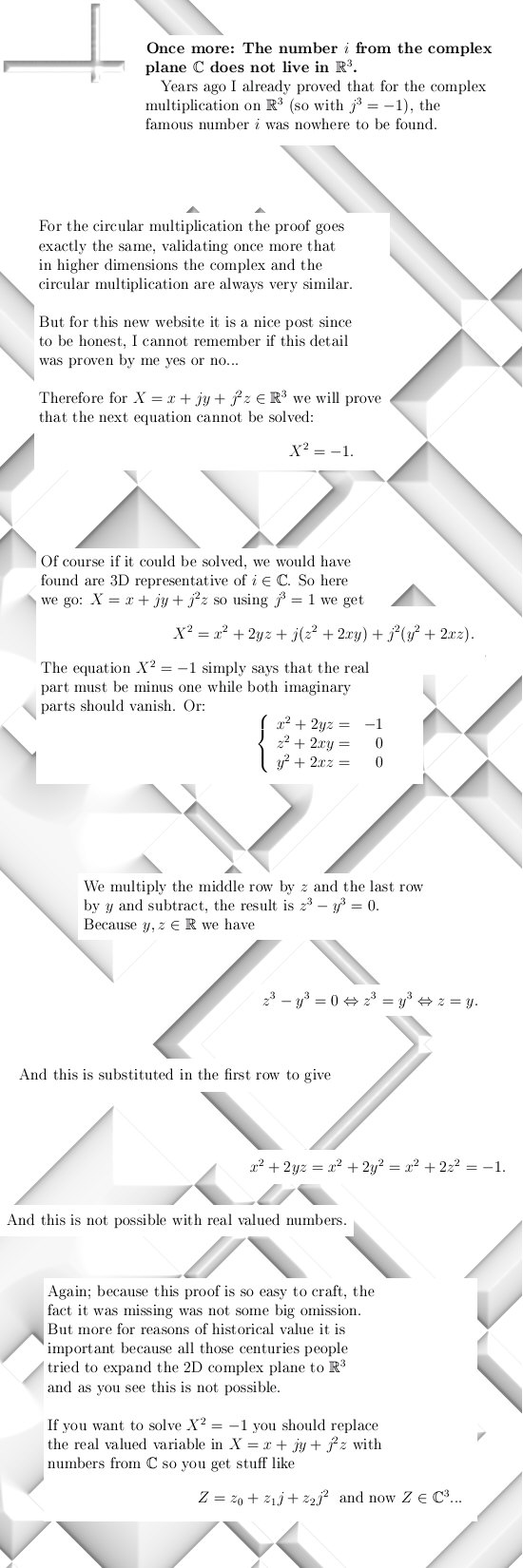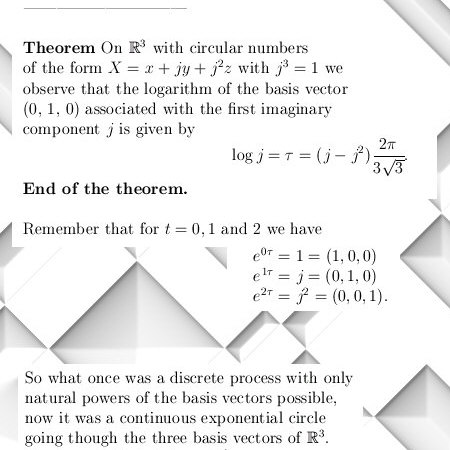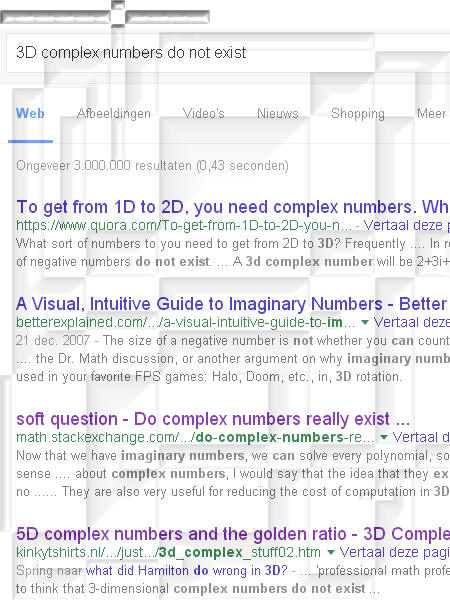# Another proof the complex number i does not live in three dimensions…

When learning about higher dimensional complex numbers, one of the things you must first understand that the complex plane as known for centuries simply does not live in three dimensional space. If you look at it from a historical perspective people always tried to start with the two dimensional complex plane and tried to expand that into three dimensions.

It does not work that way; for example you can make 21-dimensional numbers by using 3-dimensional and 7-dimensional numbers. The dimension nicely breaks down via the prime number theorem (every natural number can be uniquely written as the factors of prime numbers).

Since 2 is not a divisor of 3, it is impossible to find the complex plane in a three dimensional world…

Now years ago I proved that for the complex multiplication the number i does not exist, yet now I started this new website why not give the same proof for the circular multiplication?

Click on the picture below to read that exiting proof… 😉
(On my browser I first have to click on the picture and after that enlarge it to get the readable stuff.)Picture size is 550 pixels wide by 1650 pixels high, I tried to write it in such a way that advanced high school folks could understand it.

# Integral calculus done with matrix diagonalization.

Two days ago I posted a nice six page long update about two nasty integrals that are very hard to crack using standard math.
May be it is also possible via other methods I do not know…In the previous month I made it a math challenge to crack this nasty looking integral with the cosine stuff in it, the picture above is a so called teaser picture and it dates back to 4 Oct 2015 introducing the math challenge.

Next is a new teaser picture from two days back:Now for my old eyes it is a bit hard to read but you see some thing with e to the power tau times t in it, this is a new exponential circle just like the unit circle in the complex plane.

Well for me all this php stuff that builds this website is still very new to me, but the good thing is it can make internet pages on the fly using a database. (For example if you select a category you get all posts and pages related to that category and I do not have to make a separate page for that myself. So this should act as a strong improvement…)

Anyway here is a link to the latest update:

Integral calculus done with matrix diagonalization
http://kinkytshirts.nl/rootdirectory/just_some_math/3d_complex_stuff03.htm#21Nov2015

Ok, later this week I will take on the boring task of writing a few pages on stuff like ‘introduction to higher complex numbers’ and stuff. Now for most people higher dimensional complex numbers are a show very far away from their daily lives or from their bed so to say.
But for centuries people tried to find them, yet these people they all failed.

Back in 1990 I found them and some American guy named Dennis Morris has found them too, yet the mathematical community completely does not react at all showing the incompetence of the average professional math employee…

If you give these people a pot of gold, they think it is a pot of stolen copper.

Let’s leave it with that my dear reader, till updates.

# Ok, let’s kill the frustration.

Why was I that stupid to think it would be easily possible to craft math inside a text or html environment? Well that is because there exist websites where as a reader you comment on stuff, you can actually include math in your answer or comment…
But stuff like that is hard work, so for the time being I have to accept that I need to go on with the old way: Producing math and turn that into A4 sized pictures in the jpg format.

The setback is: when the math is all jpg pictures, no search engine can find the stuff…
So that is frustrating, on the other hand I end plenty of high enough when it comes to math and search engines; that means the old way was inefficient in speed but not in depth.

The next picture shows that on the previous website (kinkytshirts.nl) I rank about number 4 when you search for ‘3D numbers do not exist’.

Now I know a lot of stuff, but it beats me as why this update is so high in the Google search engine stuff while this is a real hardcore update related to 5D complex numbers…And going from one website to the other: pictures like the above should look like they are 450 by 600 pixels. But they don’t, ok ok I understand that in the year 2015 everything must be scaleable because all those mobile things like phones have there own screens and so.

Anyway, after the learning curve has been taken more and more posts will be added. See ya around.

__________

Useful links if you want to know a bit more about 3D complex numbers:

kinkytshirts.nl

# Introduction to 3D complex numbers.

For centuries the complex plane$\mathbb{C}$ is in use where we identify the$x$-axis with the real numbers and the$y$-axis with the imaginary numbers.

The number$i$ is the imaginary unit and for centuries we know that$i^2 = -1.$

A few centuries people have been looking to some extension of$\mathbb{C}$ to$\mathbb{R}^3$ and always they tried to have the complex plane included into the 3D real vector space. It turned out this was not possible as highlighted by a theorem known as the 2-4-8 theorem. But this theorem uses as an assumption that this extension to 3 dimensions should be bases on some quadratic form just like you can view the complex plane as generated by$z^2 = -1$.
Complex numbers are usually written as$z = x + yi.$

At present day it is generally assumed 3D complex numbers are not possible.
Yet in the year 1990 I found them, you must not use quadratic stuff in$\mathbb{R}^3$ but cubic stuff like trying to solve$X^3 = -1$
This approach gives rise to complex numbers of the form$X = x + y j + z j^2$

where if$j^3 = -1$ this is the complex multiplication and if$j^3 = 1$ this is the circular multiplication in$\mathbb{R}^3$.

In this introductory post today we only look at the complex version of stuff.

Complex numbers can be added via adding the real parts and the two corresponding imaginary parts.
Example$X = 2 + 3j + 5j^2$ and$Y = 1 + j - 4j^2$ gives the sum$X + Y = 3 + 4j + j^2.$

__________

This obviously will not work, if a very simple formulae like X = x + yj + xj^2 already does ‘not parse’ this website will never run properly. So I need to rethink a little bit; it sounded so nice you can write Latex into your posts but this is more a bucket of shit since there are two different plugin’s that fail.
End of this temporary post.

# Welcome to this ruin of a new website…

Ok, this website is supposed to be loaded with three dimensional complex number stuff in the future.

The goal is to replace all stuff as found on kinkytshirts.nl and more or less upload it into this part of the virtual space known as the internet.

So see ya later alligator.

__________$x^2+y^2=1$